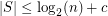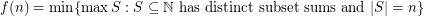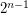# Sets with distinct subset sums

 Importance: High ✭✭✭
 Author(s):
 Subject:
 Keywords:
 Prize: $500 (Erdos-Graham)  Posted by: mdevos on: June 24th, 2007 Say that a sethas distinct subset sums if distinct subsets ofhave distinct sums. Conjecture There exists a fixed constantso thatwheneverhas distinct subset sums. Erdos valued this problem at$500, and I (M. DeVos) believe these prizes are now supported by Ron Graham.

Define the functionby the ruleThen Erdos' conjecture is equivalent to the assertion thatfor a fixed constant, and more generally, we would like to understand the behavior of.

Erdos and Moser established an upper bound on, proving that. This was later improved by a constant factor by Elkies [E].

We get an easy lower bound onby observing that the setconsisting of the firstpowers of 2 has distinct subset sums, and has maximal element. This shows that. At first glance, it might appear that such sets are optimal, but these sets have too many small numbers, and it is possible to improve upon them. Conway and Guy [CG] found a construction of sets with distinct subset sum, now called the Conway-Guy sequence, which gives an interesting upper bound on. This was this was later improved by Lunnan [L], and then by Bohman [B] to(forsufficiently large).

## Bibliography

[B] T. Bohman, A construction for sets of integers with distinct subset sums, The Electronic. Journal of Combinatorics 5 (1998) /#R3

[CG] J. H. Conway and R. K. Guy, Sets of natural numbers with distinct subset sums, Notices, Amer. Math. Soc., 15 (1968) 345.

[E] N. Elkies, An improved lower bound on the greatest element of a sum-distinct set of fixed order, J. Comb. Th. A, 41 (1986) 89-94.

[G1] R. K. Guy, Sets of integers whose subsets have distinct sums, Ann. Discrete Math., 12 (1982) 141-154.

[G2] R. K. Guy, Unsolved Problems in Number Theory, Springer-Verlag, 1981.

[L] W. F. Lunnon, Integers sets with distinct subset sums, Math. Compute, 50 (1988) 297-320.

* indicates original appearance(s) of problem.